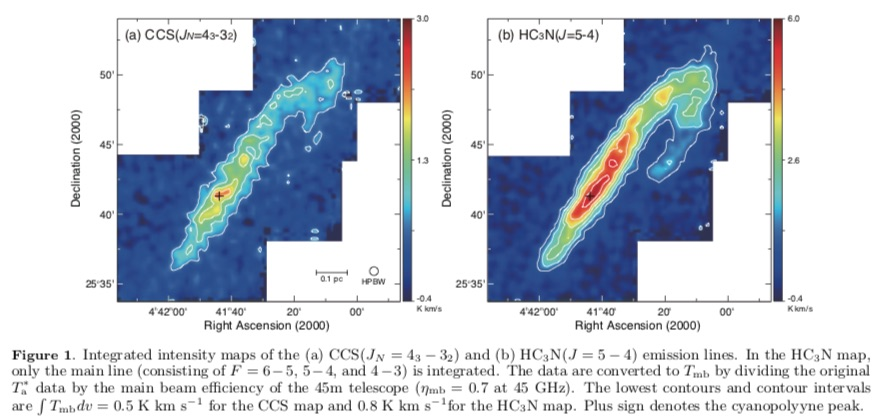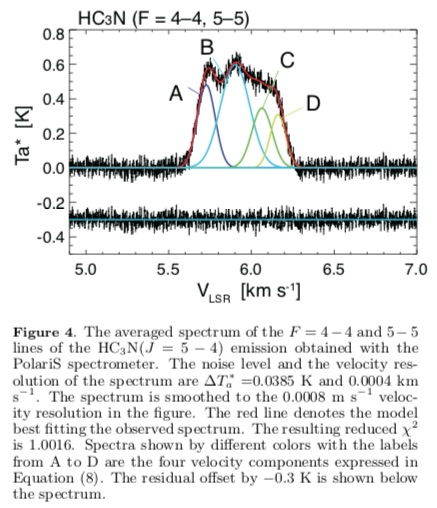# Dobashi et al. (2018) – Spectral Tomography for the Line-of-Sight Structures of the Taurus Molecular Cloud 1

We clarify the line-of-sight structure of the Taurus Molecular Cloud 1 (TMC-1) on the basis of the CCS($J_N=4_3-3_2$) and HC$_3$N($J=5-4$) spectral data observed at a very high velocity resolution and sensitivity of $\Delta V \simeq 0.0004$ km s$^{-1}$ ($=61$ Hz) and $\Delta T_{\rm mb} \simeq 40$ mK. The data were obtained toward the cyanopolyyne peak with $\sim$30 hours integration using the Z45 receiver and the PolariS spectrometer installed in the Nobeyama 45m telescope. Analyses of the optically thin $F=4-4$ and $5-5$ hyperfine lines of the HC$_3$N emission show that the spectra consist of four distinct velocity components with a small line width ($\lesssim 0.1$ km s$^{-1}$) at $V_{\rm LSR}=$5.727, 5.901, 6.064, and 6.160 km s$^{-1}$, which we call A, B, C, and D, respectively, in the order of increasing LSR velocities. Utilizing the velocity information of the four velocity components, we further analyzed the optically thicker CCS spectrum and the other hyperfine lines of the HC$_3$N emission by solving the radiative transfer to investigate how the four velocity components overlap along the line of sight. Results indicate that they are located in the order of A, B, C, and D from far side to near side to the observer, indicating that TMC-1 is shrinking, moving inward as a whole.

Dobashi, Kazuhito; Shimoikura, Tomomi; Nakamura, Fumitaka; Kameno, Seiji; Mizuno, Izumi; Taniguchi, Kotomi
2018, ArXiv e-prints, 1808, arXiv:1808.01802